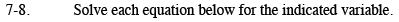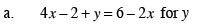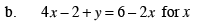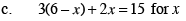### Home > AC > Chapter 7 > Lesson 7.1.1 > Problem7-8

7-8.
1. Solve each equation below for the indicated variable. Homework Help ✎

1. 4x − 2 + y = 6 − 2x for y

2. 4x − 2 + y = 6 − 2x for x

3. 3(6 − x) + 2x = 15 for x4x + y = 8 − 2x

Subtract 4x from both sides.

y = 8 − 6xFollow the steps in part (a).

$x = -\frac{1}{6}y+\frac{8}{6}$Multiply to remove the parentheses then follow the steps in part (a).